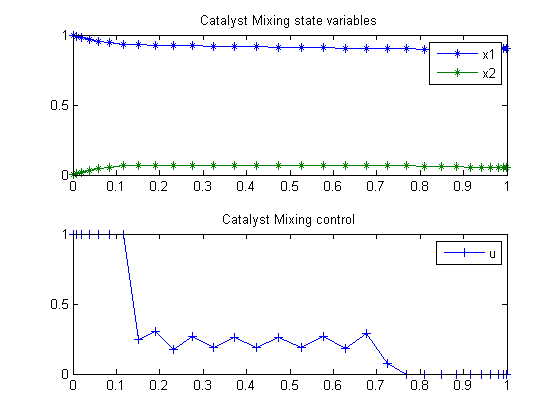22  Catalyst Mixing

Second-order sensitivities of general dynamic systems with application to optimal control problems. 1999, Vassilios S. Vassiliadis, Eva Balsa Canto, Julio R. Banga

Case Study 6.2: Catalyst mixing

22.1  Problem formulation

This problem considers a plug-flow reactor, packed with two catalysts, involving the reactions

S1 <-> S2 -> S3

The optimal mixing policy of the two catalysts has to be determined in order to maximize the production of species S3. This dynamic optimization problem was originally proposed by Gunn and Thomas (1965), and subsequently considered by Logsdon (1990) and Vassiliadis (1993). The mathematical formulation is

Maximize:

 J = 1 − x1(tf) − x2(tf)

subject to:

 dx1 dt
= u*(10*x2x1
 dx2 dt
= u*(x1−10*x2)−(1−u)*x2
 0 <= u <= 1
 x(t0) = [1  0]′
 tf = 1

Reference: 

22.2  Problem setup

toms t
p = tomPhase('p', t, 0, 1, 30);
setPhase(p);

tomStates x1 x2
tomControls u

% Initial guess
% Note: The guess for t_f must appear in the list before expression involving t.
x0 = {icollocate({
x1 == 1-0.085*t
x2 == 0.05*t
})
collocate(u==1-t)};

% Box constraints
cbox = {0.9 <= icollocate(x1) <= 1
0 <= icollocate(x2) <= 0.1
0 <= collocate(u)   <= 1};

% Boundary constraints
cbnd = {initial({x1 == 1; x2 == 0})
final({x1 <= 0.95})};

% ODEs and path constraints
ceq = collocate({
dot(x1) == u.*(10*x2-x1)
dot(x2) == u.*(x1-10*x2)-(1-u).*x2});

% Objective
objective = -1+final(x1)+final(x2);

22.3  Solve the problem

options = struct;
options.name = 'Catalyst Mixing';
solution = ezsolve(objective, {cbox, cbnd, ceq}, x0, options);
t  = subs(collocate(t),solution);
x1 = subs(collocate(x1),solution);
x2 = subs(collocate(x2),solution);
u  = subs(collocate(u),solution);
Problem type appears to be: lpcon
Starting numeric solver
===== * * * =================================================================== * * *
TOMLAB - Tomlab Optimization Inc. Development license  999001. Valid to 2011-02-05
=====================================================================================
Problem: ---  1: Catalyst Mixing                f_k      -0.048059280695325390
sum(|constr|)      0.000000452031812690
f(x_k) + sum(|constr|)     -0.048058828663512701
f(x_0)      0.964999999999998080

Solver: snopt.  EXIT=0.  INFORM=1.
SNOPT 7.2-5 NLP code
Optimality conditions satisfied

FuncEv    1 ConstrEv   66 ConJacEv   66 Iter   43 MinorIter  248
CPU time: 0.171875 sec. Elapsed time: 0.188000 sec.

22.4  Plot result

subplot(2,1,1)
plot(t,x1,'*-',t,x2,'*-');
legend('x1','x2');
title('Catalyst Mixing state variables');

subplot(2,1,2)
plot(t,u,'+-');
legend('u');
title('Catalyst Mixing control');